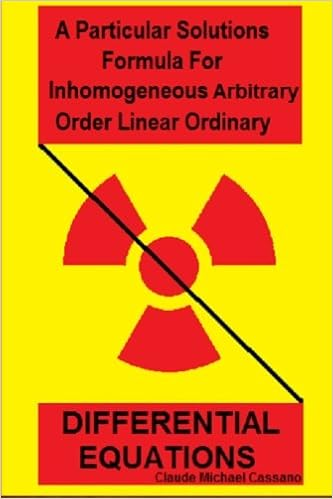## A Particular Solutions Formula For Inhomogeneous Arbitrary Order Linear Ordinary Differential Equations

#### Abstract

A particular solution for any nonhomogeneous linear second, third, and fourth order ordinary differential equation is generally determined. Applying what was determined thus; and following by example a particular solution formula for arbitrary order is obtained. Finding a particular solutions to a linear nonhomogeneous ordinary differential equation has always been a process of determining homogeneous solutions, and then adding any particular solution of the nonhomogeneous equation. The well-known methods of undetermined coefficients and variation of parameters have long been the standard in determining this particular solution. The former has sometimes been considered 'ad-hoc', and both can be intricate. A relatively simple formula has been found which allows the particular solution to be written and evaluated immediately.#### Cite this work

Researchers should cite this work as follows:

• CLAUDE MICHAEL CASSANO (2017), "A Particular Solutions Formula For Inhomogeneous Arbitrary Order Linear Ordinary Differential Equations," https://simiode.org/resources/3581.